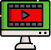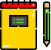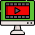VideosNotes

### Quadratic Equations. (A) - 1

A quadratic equation is an equation of the second degree, meaning it contains at least one term that is squared. The standard form is ax² + bx + c = 0 with a, b, and c being constants, or numerical coefficients, and x is an unknown variable.
Views: 3
Video Duration: 11:56 (Part 1 of 4)